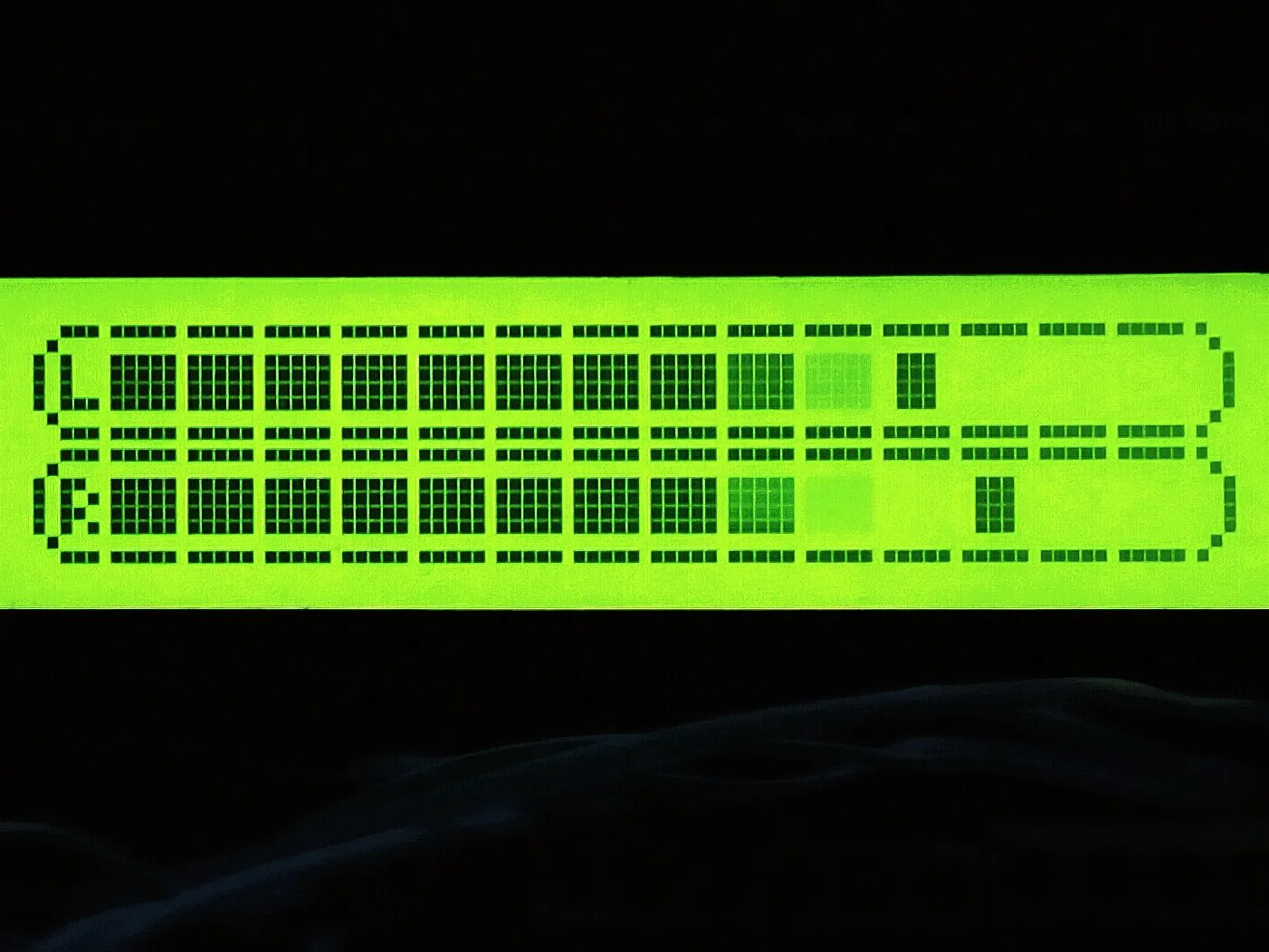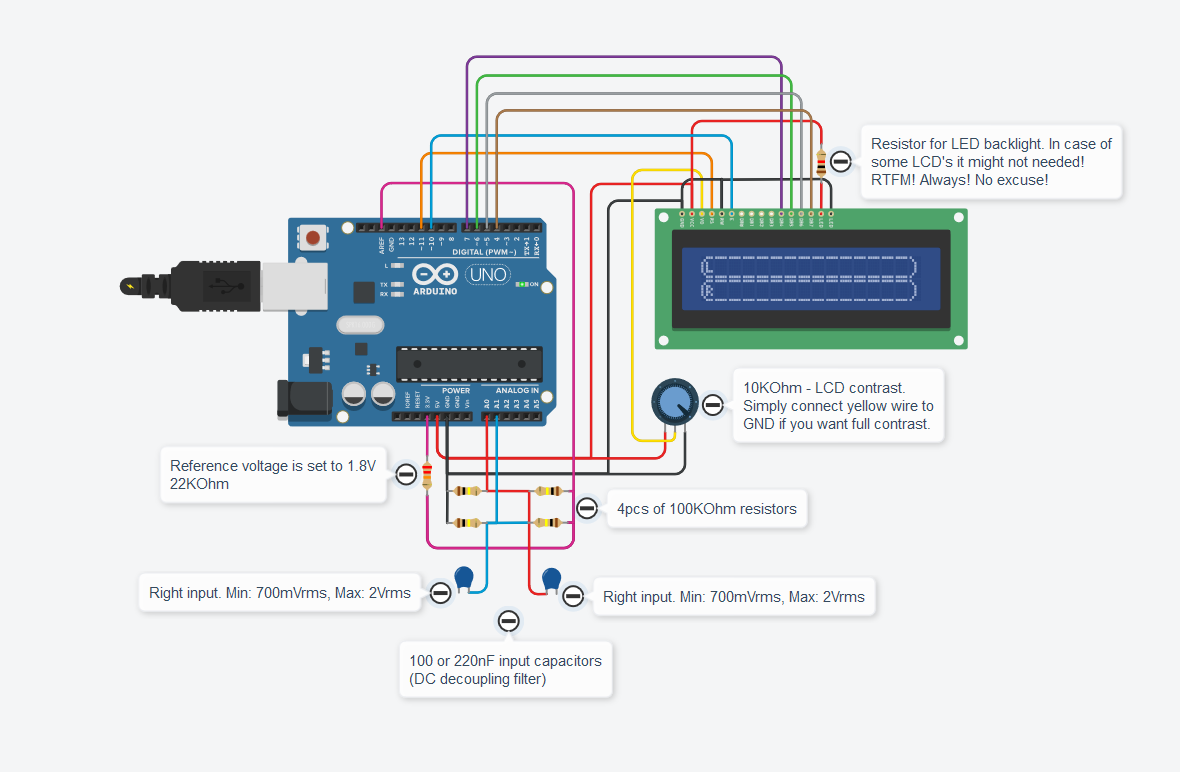# KTAudio VU Meter © GPL3+

Simple, easy to build 2 x 14 bars stereo VU meter for representing audio level.

• 13,198 views
• 17 respects

## Necessary tools and machinesSoldering iron (generic)

## Apps and online services

First of all, this project is aiming to entertain those who like the digital VU (Volume Unit) meters and want to build their own version. The project is easy to build, no deep skills required. However, for better sound vs level visual representation you would need some soldering skills. The input level shifter circuit and DC decouplers are required some attention. Please bear in mind that this device is intended to use this way with low-level audio signals. ( < 2Vrms!)

The only thing you need to create is to create the left and right level shifter circuit and attach it to your Arduino board. Then load the sourcecode and you are almost ready.

Please note that you might need to use separated power supply (separated from your PC) to avoid any kind of ground loop situation with your hifi / pa system! Since Arduino is a sensitive device and lacks of any surge protection, you need to be cautious with ground looping issue.

In the below video you can see it's very simple design: Of course, you can build it with I2C LCD driver as well to reduce the required amount of wires.

• Easy to build
• Fairly accurate representation of sound level
• Very good entertaining and for learning purposes
• Can be driven with low level of audio
• It is easy to add / attach to existing PA or HiFi system

• Linear representation of the volume level (From around -14dB to 0dB)
• Not accurate enough for true representation for studio or usage where accuracy is essential
• High update rate can cause screen flickering
• Low update rate can cause delay in representation

In the next project I will share another source code that has no disadvantages like this version. ArVUmeter 1.0 will be shared soon...

## Code

##### Main.cpp.inoArduino
```/*
Arduino based VU meter by KTAudio.
Developed by ThomAce (Tamas Kamocsai) based on siemenwauters, theredstonelabz and michiel H's VU meter.

Developer: ThomAce (Tamas Kamocsai)
Mail: thomacepcg@gmail.com
Version: 1.0
Last modification date: 2019.09.24

Original version:
https://www.instructables.com/id/ARDUINO-VU-METER/

Original description:
VU meter by siemenwauters, theredstonelabz and michiel H don't forget to like and subscribe to support my work. tnx
*/

#include <LiquidCrystal.h>

byte Bar = {
B11111,
B00000,
B11111,
B11111,
B11111,
B11111,
B00000,
B11111
};

byte L = {
B00111,
B01000,
B10100,
B10100,
B10100,
B10111,
B01000,
B00111
};

byte R = {
B00111,
B01000,
B10110,
B10101,
B10110,
B10101,
B01000,
B00111
};

byte EndMark = {
B10000,
B01000,
B00100,
B00100,
B00100,
B00100,
B01000,
B10000
};

byte EmptyBar = {
B11111,
B00000,
B00000,
B00000,
B00000,
B00000,
B00000,
B11111
};

byte peakHoldChar = {
B11111,
B00000,
B01110,
B01110,
B01110,
B01110,
B00000,
B11111
};

String main_version = "1.0";
int left, right;                        //Variables to store and calculate the channel levels
const int numReadings = 5;              //Refresh rate. Lower value = higher rate. 5 is the defaul
int indexL = 0;                         //Actual channel index
int totalL = 0;                         //Total channel data
int maxL = 0;                           //Maximal level
int indexR = 0;
int totalR = 0;
int maxR = 0;
int inputPinL = A1;                     //Input pin Analog 1 for LEFT channel
int inputPinR = A0;                     //Input pin Analog 0 for RIGHT channel
int volL = 0;
int volR = 0;
int rightAvg = 0;
int leftAvg = 0;
long peakHoldTime = 1500;               //peak hold time in miliseconds
long peakHold = 0;
int rightPeak = 0;
int leftPeak = 0;
long decayTime = 0;
long actualMillis = 0;

LiquidCrystal lcd(11, 10, 7, 6, 5, 4);  //lcd configuration

void setup()
{
lcd.begin(40, 2); //Setting up LCD. 16 chars and 2 rows

lcd.createChar(1, Bar);
lcd.createChar(2, L);
lcd.createChar(3, R);
lcd.createChar(4, EmptyBar);
lcd.createChar(5, EndMark);
lcd.createChar(6, peakHoldChar);

String KTAudio = "KTaudio project";

for (int i = 0; i <= 16; i++)
{
lcd.setCursor(0, 0);
lcd.print(KTAudio.substring(0, i));
delay(50);
}

KTAudio = "VU meter " + main_version;

for (int i = 0; i <= KTAudio.length(); i++)
{
lcd.setCursor(0, 1);
lcd.print(KTAudio.substring(0, i));
delay(50);
}

delay(500);

lcd.clear();
lcd.setCursor(0, 0);

for (int i = 0; i < 16; i++)
{
lcd.setCursor(i, 1);
lcd.write(4);
}

for (int i = 0; i < 16; i++)
{
lcd.setCursor(i, 1);
lcd.write(1);

delay(50);
}

delay(500);
lcd.clear();

decayTime = millis();
}

void loop()
{
actualMillis = millis();

lcd.setCursor(0, 0);        //L channel index
lcd.write(2);               //L symbol
lcd.setCursor(0, 1);        //R channel index
lcd.write(3);               //R symbol
lcd.setCursor(15, 0);       //closing tag / end mark index 1
lcd.write(5);               //closing tag / end mark
lcd.setCursor(15, 1);       //closing tag / end mark index 2
lcd.write(5);               //closing tag / end mark

totalL = analogRead(inputPinL) / 4 - 128; //reducing the detected hum and noise

if(totalL > maxL)
{
maxL = totalL;
}

indexL++;

{
indexL = 0;
left = maxL;
maxL = 0;
}

totalR = analogRead(inputPinR) / 4 - 128; //reducing the detected hum and noise

if(totalR > maxR)
{
maxR = totalR;
}

indexR++;

{
indexR = 0;
right = maxR;
maxR = 0;
}

volR = right / 3;

if(volR > 14)
{
volR = 14;
}

if (volR < (rightAvg - 2))
{
if (decayTime < actualMillis)
rightAvg--;

volR = rightAvg;
}
else if (volR > (rightAvg + 2))
{
volR = (rightAvg + 2);
rightAvg = volR;
}
else
{
rightAvg = volR;
}

if (volR > rightPeak)
{
rightPeak = volR;
}

drawBar(volR, rightPeak, 1);

volL = left / 3;

if(volL > 14)
{
volL = 14;
}

if (volL < (leftAvg - 2))
{
if (decayTime < actualMillis)
leftAvg--;

volL = leftAvg;
}
else if (volL > (leftAvg + 2))
{
volL = (leftAvg + 2);
leftAvg = volL;
}
else
{
leftAvg = volL;
}

if (volL > leftPeak)
{
leftPeak = volL;
}

drawBar(volL, leftPeak, 0);

if (decayTime < actualMillis)
decayTime = (millis() + 50);

if (peakHold < actualMillis)
{
peakHold = (millis() + peakHoldTime);
rightPeak = -1;
leftPeak = -1;
}
}

void drawBar(int data, int peakData, int row)
{
//If the previous peak data is 1 or 0, then not taking care of the value.
if (peakData < 2)
{
peakData = -1;
}

//First char (idx 0) = R or L
//Last (16th) char (idx 15) is the closing mark of the bar.
//We have 14 chars to write.
for (int col = 1; col < 15; col++)
{
lcd.setCursor(col, row);

if (col < data)
{
lcd.write(1); //write bar element
}
else if (peakData == col)
{
lcd.write(6); //write the peak marker
}
else
{
lcd.write(4); //write "empty"
}
}
}
```

## Schematics#### Published on

September 24, 2019

#### Members who respect this project

See similar projects
you might like

#### VU Meter for Audio Signal (dBu) using LCD

Project tutorial by deltakilo

• 6,299 views
• 6 respects

#### Stereo NeoPixel Ring VU Meter

Project showcase by ericBcreator

• 115,919 views
• 206 respects

#### Network VU Sound Level Meter using C#, Arduino and ESP8266

Project tutorial by Fanatic Series

• 3,190 views
• 7 respects

#### 2 x 16-Band Audio Spectrum Analyzer with LCD

by shajeeb and ThomAce

• 11,849 views
• 12 respects

#### Infinity Mirror VU Meter Music Equalizer

Project showcase by TheTNR

• 13,199 views